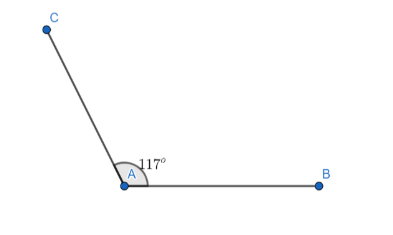Courses
Courses for Kids
Free study material
Free LIVE classes
MoreLIVE
Join Vedantu’s FREE Mastercalss

# What is an Obtuse Angle?Verified
362.4k+ views
Hint: The given question is related to types of angles. Try to recall the various types of angles, their definition, and their examples.

In mathematics, an angle is defined as the measure of inclination of one line (or line segment) on another line (or line segment). Based on the measure of the angle, angles are classified into six types:
Acute angle
Right angle
Obtuse angle
Straight angle
Reflex angle
Complete angle (or Full angle)
Acute angle: Any angle whose measure is more than ${{0}^{o}}$ and less than ${{90}^{o}}$ is called an acute angle.
Right angle: The angle whose measure is exactly ${{90}^{o}}$ is called a right angle.
Straight angle: The angle whose measure is exactly ${{180}^{o}}$ is called a straight angle.
Reflex angle: Any angle whose measure is more than ${{180}^{o}}$ and less than ${{360}^{o}}$ is called a reflex angle.
Complete angle: The angle whose measure is exactly ${{360}^{o}}$ is called a complete angle.
Obtuse angle: Any angle whose measure is more than ${{90}^{o}}$ and less than ${{180}^{o}}$ is called an obtuse angle. For example : ${{95}^{o}},{{111}^{o}},{{133}^{o}}$ , etc.
The figure of an obtuse angle is shown below:The measure of the angle in the figure is equal to ${{117}^{o}}$ , which lies between ${{90}^{o}}$ and ${{180}^{o}}$ . Hence, it is an obtuse angle.

Note: Students are generally confused between obtuse angles and reflex angles. Obtuse angles are those angles whose measure is more than ${{90}^{o}}$ and less than ${{180}^{o}}$, whereas, reflex angles are those angles whose measure is more than ${{180}^{o}}$ and less than ${{360}^{o}}$. Such confusion should be avoided.
Last updated date: 22nd Sep 2023
Total views: 362.4k
Views today: 5.62k